# How to use SUMIF function in Excel with formula examples

This tutorial explains the Excel SUMIF function in plain English. The main focus is on real-life formula examples with all kinds of criteria including text, numbers, dates, wildcards, blanks and non-blanks.

Microsoft Excel has a handful of functions to summarize large data sets for reports and analyses. One of the most useful functions that can help you make sense of an incomprehensible set of diverse data is SUMIF. Instead of adding up all numbers in a range, it lets you sum only those values that meet your criteria.

So, whenever your task requires conditional sum in Excel, the SUMIF function is what you need. A good thing is that the function is available in all versions, from Excel 2000 through Excel 365. Another great thing is that once you've learned SUMIF, it will take you very little effort to master other "IF" functions such as SUMIFS, COUNTIF, COUNTIFS, AVERAGEIF, etc.

## SUMIF in Excel - syntax and basic uses

The SUMIF function, also known as Excel conditional sum, is used to add up cell values based on a certain condition.

The function is available in Excel 365, Excel 2021, Excel 2019, Excel 2016, Excel 2013, Excel 2010, Excel 2007, and lower.

The syntax is as follows:

SUMIF(range, criteria, [sum_range])

As you see, the SUMIF function has 3 arguments - first 2 are required and the last one is optional.

• Range (required) - the range of cells to be evaluated by criteria.
• Criteria (required) - the condition that must be met. It may be supplied in the form of a number, text, date, logical expression, a cell reference, or another Excel function. For example, you can enter the criteria such as "5", "cherries", "10/25/2014", "<5", etc.
• Sum_range (optional) - the range to sum if the condition is met. If omitted, then range is summed.

Note. Please pay attention that any text criteria or criteria containing logical operators must be enclosed in double quotation marks, e.g. "apples", ">10". Cell references should be used without the quotation marks, otherwise they would be treated as text strings.

## Basic SUMIF formula

To better understand the SUMIF syntax, consider the following example. Suppose you have a list of products in column A, regions in column B, and sales amounts in column C. Your goal is to get a total of sales for a specific region, say North. To have it done, let's build an Excel SUMIF formula in its simplest form.

• Range - a list of regions (B2:B10).
• Criteria - "North" or a cell containing the region of interest (F1).
• Sum_range - the sales amounts to be added up (C2:C10).

Putting the arguments together, we get the following formula:

`=SUMIF(B2:B10, "north", C2:C10)`

or

`=SUMIF(B2:B10, F1, C2:C10)`

Both formulas only sum sales in the North region: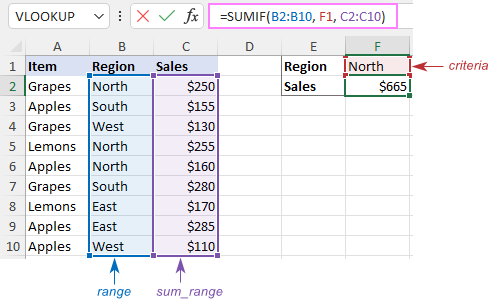Note. The sum_range parameter actually defines only the upper leftmost cell of the range to be summed. The remaining area is defined by the dimensions of the range argument. In practice, this means that sum_range argument does not necessarily have to be of the same size as range argument, i. e. it may have a different number of rows and columns. However, the top left cell must always be the right one. For example, in the above formula, you can supply C2, or C2:C4, or even C2:C100 as the sum_range argument, and the result will still be correct. However, the best practice is to provide equally sized range and sum_range.

Note. The SUMIF function is case-insensitive by nature. However, it is possible to force it to recognize the text case. For full details, please see Case-sensitive SUMIF in Excel.

## How to use SUMIF in Excel - formula examples

Hopefully, the above example has helped you gain some basic understanding of how the function works. Below you will find a few more formulas that demonstrate how to use SUMIF in Excel with various criteria.

### SUMIF greater than or less than

To sum numbers greater than or less than a particular value, configure the SUMIF criteria with one of the following logical operators:

• Greater than (>)
• Greater than or equal to (>=)
• Less than (<)
• Less than or equal to (<=)

In the table below, supposing you wish to add up the sales numbers for the items that ship in 3 or more days. To express this condition, put a comparison operator (>) before the number and surround the construction in double quotes:

`=SUMIF(C2:C10, ">3", B2:B10)`

If the target number is in another cell, say F1, concatenate the logical operator and cell reference:

`=SUMIF(C2:C10, ">"&F1, B2:B10)`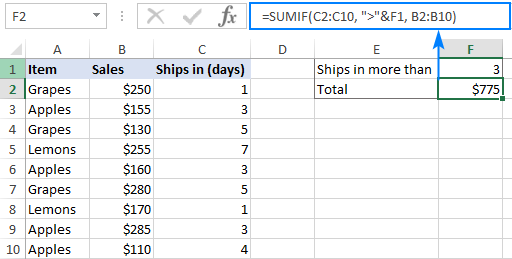In a similar manner, you can sum values smaller than a given number. For this, use the less than (<) operator:

`=SUMIF(C2:C10, "<3", B2:B10)`

### SUM IF equal to

A SUMIF formula with the "equal to" criteria works for both numbers and text. In such criteria, the equals sign is not actually required.

For instance, to find a total of the items that ship in 3 days, either of the below formulas will do:

`=SUMIF(C2:C10, 3, B2:B10)`

or

`=SUMIF(C2:C10, "=3", B2:B10)`

To sum if equal to cell, supply a cell reference for criteria:

`=SUMIF(C2:C10, F1, B2:B10)`

Where B2:B10 are the amounts, C2:C10 is the shipment duration, and F1 is the desired delivery time.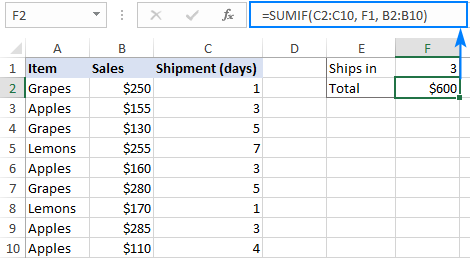Likewise, you can use the "equal to" criteria with text values. For instance, to add up the Apples amounts, choose any of the formulas below:

`=SUMIF(A2:A10, "apples", B2:B10)`

`=SUMIF(A2:A10, "=apples", B2:B10)`

`=SUMIF(A2:A10, F1, B2:B10)`

Where A2:A10 is the list of items to compare against the value in F1.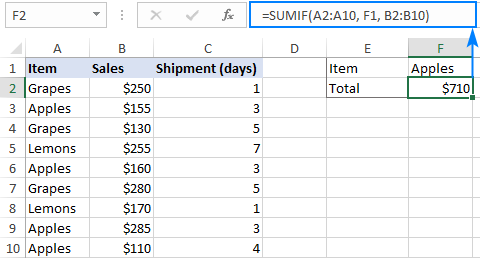The above formulas imply that the criterion matches the entire cell contents. Consequently, the SUMIF function will add up Apples sales but not, say, Green Apples. To sum partial matches, construct the "if cell contains" criteria like in this SUMIF wildcard formula.

Note. Please pay attention that, in Excel SUMIF formulas, a comparison or equals operator should always be enclosed in double quotes, whether used on its own or together with a number or text.

### SUM IF not equal to

To build the "not equal to" criteria, use the "<>" logical operator.

When a value, either text or number, is hardcoded in the criteria, remember to surround the entire construction with double quotes.

For example, to sum the amounts with shipment other than 3 days, the formula goes as follows:

`=SUMIF(C2:C10, "<>3", B2:B10)`

To find a total of all the items except Apples, the formula is:

`=SUMIF(A2:A10, "<>apples", B2:B10)`

When the criterion is in another cell, concatenate the "not equal to" operator and a cell reference like this:

`=SUMIF(A2:A10, "<>"&F1, B2:B10)`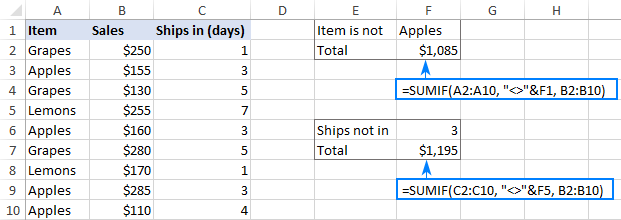### SUM IF blank

This example shows how to sum cells in one column if a corresponding cell in another column is blank. There are two formulas to fulfill the task. Which one to use depends on your interpretation of a "blank cell".

If "blank" means cells that contain absolutely nothing (no formula, no zero-length string returned by some other function), then use "=" for criteria. For example:

`=SUMIF(B2:B10, "=", C2:D10)`

If "blank" includes empty strings (for example, cells with a formula like =""), then use "" for criteria:

`=SUMIF(B2:B10, "", C2:D10)`

Both formulas return a total of sales for undefined regions, i.e. where a cell in column B is blank: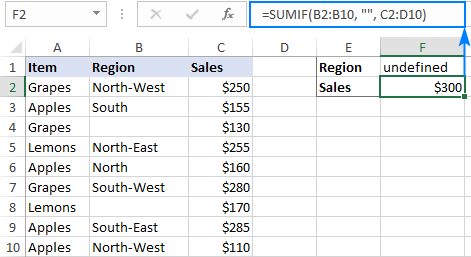### SUM IF not blank

To make "if cell is not blank then sum" kind of formula, use "<>" as the criteria. This will add up all cells that contain anything in them, including zero-length strings.

For instance, here's how you can sum sales for all the regions, i.e. where column B is not blank:

`=SUMIF(B2:B10, "<>", C2:D10)`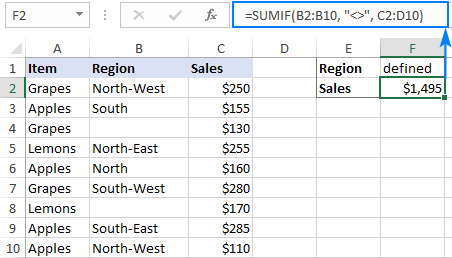### Excel SUMIF with text criteria

When adding up numbers in one column based on text values in another column, it's important to differentiate between exact and partial match.

Criteria Formula Example Description
Sum if equal to Exact match:
=SUMIF(A2:A8, "bananas", C2:C8)
Sum values in cells C2:C8 if a cell in column A in the same row contains exactly the word "bananas" and no other words or characters. Cells containing "green bananas", "bananas green", or "bananas!" are not included.
Sum if cell contains Partial match:
=SUMIF(A2:A8, "*bananas*", C2:C8)
Sum values in cells C2:C8 if a corresponding cell in column A contains the word "bananas", alone or in combination with any other words. Cells containing "green bananas", "bananas green", or "bananas!" are summed.
Sum if not equal to Exact match:
=SUMIF(A2:A8, "<>bananas", C2:C8)
Sum values in cells C2:C8 if a cell in column A contains any value other than "bananas". If a cell contains "bananas" together with some other words or characters like "yellow bananas" or "bananas yellow", such cells are summed.
Sum if cell does not contain Partial match:
=SUMIF(A2:A8, "<>*bananas*", C2:C8)
Sum values in cells C2:C8 if a cell in column A does not contain the word "bananas", alone or in combination with any other words. Cells containing "yellow bananas" or "bananas yellow" are not summed.

For real-life formula examples, please check out Sum if equal to and Sum if not equal to.

In the next section, we'll take a closer look at SUMIF formulas with partial match.

## SUMIF formulas with wildcard characters

To conditionally sum cells by partial match, include one of the following wildcard characters in your criteria:

• Question mark (?) to match any single character in a specific position.
• Asterisk (*) to match any number of characters.

### Example 1. Sum values based on partial match

Suppose you wish to total sales for all northern regions, including North, North-East, and North-West. To have it done, put an asterisk right after the word "north":

`=SUMIF(B2:B10, "north*", C2:D10)`

An asterisk on both sides will also work:

`=SUMIF(B2:B10, "*north*", C2:D10)`

Alternatively, you can type the region of interest in a predefined cell (F1), and then concatenate a cell reference and a wildcard character enclosed in quotes:

`=SUMIF(B2:B10, F1&"*", C2:D10)`

Or

`=SUMIF(B2:B10, "*"&F1&"*", C2:D10)`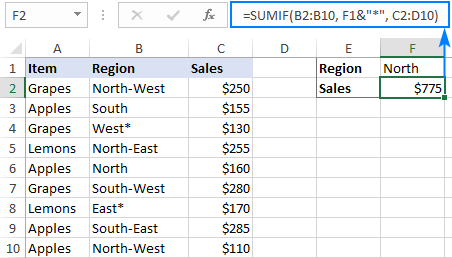### Example 2. Sum if cell contains * or ?

To match a literal question mark or asterisk, place a tilde (~) before the character, e.g. "~?" or "~*".

For example, to sum sales for the regions marked with *, use "*~*" for criteria. In this case, the first asterisk is a wildcard and the second one is a literal asterisk character:

`=SUMIF(B2:B10, "*~*", C2:D10)`

If the criteria (* in our case) is entered in a separate cell, then concatenate a tilde and the cell reference, like this:

`=SUMIF(B2:B10, "*"&"~"&F1, C2:D10)`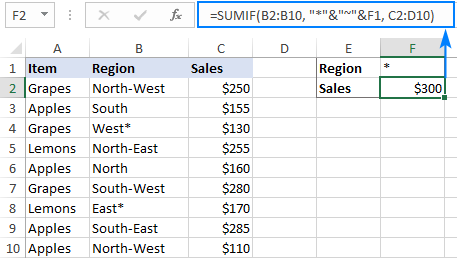### Example 3. Sum if another cell contains text

If your dataset contains various data types and you only want to sum cells corresponding to text values, the following SUMIF formulas will come in handy.

To add up values in cells C2:C8 if a cell in column A contains any text character(s):

`=SUMIF(A2:A8,"?*", C2:C8)`

To sum values in C2:C8 if a cell in column A contains any text value, including zero length strings:

`=SUMIF(A2:A8,"*", C2:C8)`

Both of the above formulas ignore non-text values such as numbers, dates, errors, and Booleans.

## How to use Excel SUMIF with dates

Using dates as SUMIF criteria is very much like using numbers. The most important thing is to supply a date in the format that Excel understands. If you are not sure which date format is supported and which is not, the DATE function can be a solution.

Assuming you are looking to sum sales for the items delivered before 10-Sep-2020, the criteria can be expressed in this way:

`=SUMIF(C2:C10, "<9/10/2020", B2:B10)`

or

`=SUMIF(C2:C10, "<"&DATE(2020,9,10), B2:B10)`

or

`=SUMIF(C2:C10, "<"&F1, B2:B10)`

Where F1 is the target date.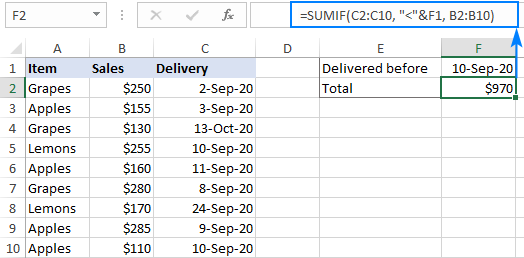To sum cells based on today's date, include the TODAY function in your criteria. For example, that's how you calculate a total of sales with a delivery date prior to today:

`=SUMIF(C2:C10, "<"&TODAY(), B2:B10)`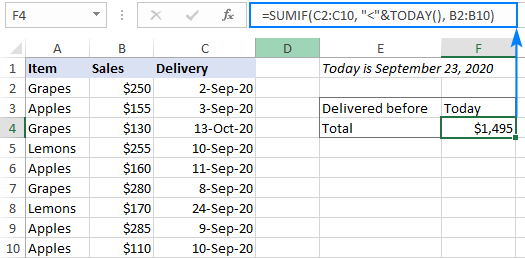To sum within a date range, you need to define a smaller and larger date separately. This can be done with the help of the SUMIFS function that supports multiple criteria.

For example, to sum values in column B if a date in column C is between two dates, this is the formula to use:

`=SUMIFS(B2:B10, C2:C10, ">="&F1, C2:C10, "<="&G1)`

Where B2:B10 is the sum range, C2:C10 is the list of dates to check, F1 is the start date and G1 is the end date.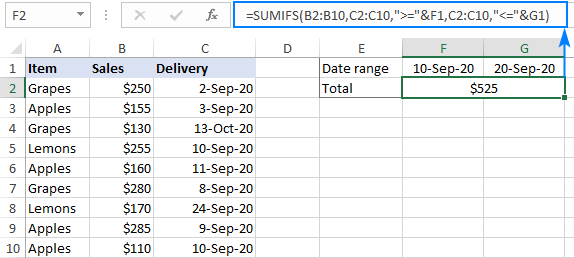More formula examples can be found in SUMIFS with date range as criteria.

## How to do SUMIF from another sheet

To conditionally sum data from a different sheet, provide external references for the SUMIF arguments. The easiest way is to start typing a formula, switch to another worksheet and select ranges using the mouse. Excel will insert all the references automatically, without you having to worry about the correct syntax.

For instance, the below formula will add up values in C2:C10 on the Data sheet based on the criteria in B3 on Sheet1:

`=SUMIF(Data!B2:B10, B3, Data!C2:C10)`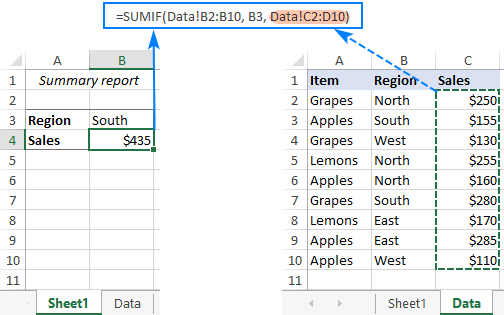To create a flexible formula, you normally insert all variable parameters in predefined cells instead of "hardcoding" them. With Excel SUMIF, that might be a bit of a challenge.

In the simplest case when summing "if equal to", you simply use a cell reference for criteria. For example:

`=SUMIF(C2:C10, F1, B2:B10)`

But when a cell reference is used together with a logical operator, the criteria should be provided in the form of a string. So, you use the double quotes ("") to start a text string and ampersand (&) to concatenate and finish the string off. For example:

`=SUMIF(C2:C10, ">"&F7, B2:B10)`

Please note that the comparison operators are enclosed in quotation marks while the cell references are not.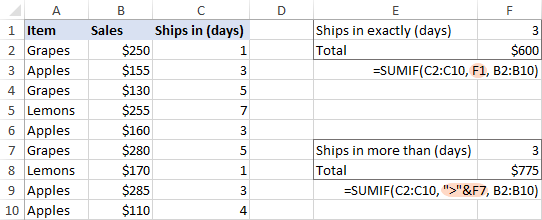1. I am trying to build a formula uses cells for the criteria that is part of sumif function. For example.
F20 = '[C2:C23]
F19 = 'A2:A23

c20 = sumif(F19,A22,F20)

Please let me know what I'm doing wrong.

thanks
Don

• Hi! Only references to data cells and criterion values can be arguments of the SUMIF function. Read carefully the article above.

2. On Sheet1 in SmartSheet I have:
- a Project Reference column which could include whole numbers (i.e. 4) to identify Parent projects or decimals (i.e. 4.1, 4.2, 4.3) to identify sub-projects within the parent project. Eventually this project reference column could contain numbers from 1 to 100.
- a Project column which lists the project name and/or any sub-project names as children tasks
- a Total Actuals Sub-Project column which uses the following formula: "=SUMIFS({Sheet2 FY24 YTD}, {Sheet2 Project Ref}, [Project Reference]@row, {Sheet2 Res/Act}, "ACTUALS")"
- a Total Actual per Project column: the formula for this is where I'm having trouble. See explanation further below.

On Sheet2 in SmartSheet I have:
- a Project Reference column (this column is manually updated with the associated project reference assign from Sheet1
- a Reserve/Actual column in which either Reserve or Actuals is selected
- a Project column that uses the following formula to auto-populate the exact project name from Sheet1: "=INDEX({Sheet1 Range 1: Project}, MATCH([Project Reference]@row, {Sheet1 Range 1: Project Ref}, 0))"
- a FY24 YTD column which SUMS designated cells

I'm having trouble creating the formula for Total Actual per Project column on Sheet1. What I'd like it to do is look at the parent row - in this case the Project Reference # is 4, with 4.1, 4.2, 4.3 as sub-projects (it's important to note here that not all projects have sub-projects) - and add the values from Total Actuals Sub-project column IF the value in the Project Reference column is >= 4 AND <=4.999.

Thank you!

• Hi! Unfortunately, I could not understand your problem. Try to describe it more shortly, give an example of source data and desired result.

3. Hello,

I am totalling numbers from column A based on criteria in column B.
But I need the numbers from column A only to be totalled if they are between \$25,001 and \$50,000. How do i add this condition.

Essentially i need to merge these to formulars.

=SUMIF(\$K\$3:\$K\$1129,P1,\$E\$3:\$E\$1129)
=SUMIFS(E:E,E:E,">"&P24,E:E,"<"&P25)

• Hi! Use the SUMIFS function and add all the conditions to this formula. For example:

=SUMIFS(\$E\$3:\$E\$1129,\$K\$3:\$K\$1129,P1,\$E\$3:\$E\$1129,">"&P24,\$E\$3:\$E\$1129,"<"&P25)

4. Hello, can we automatically sort column in the criteria without put the criteria one by one into the formula. Example i have 60 criteria that want to sum up, how exactly we can simply sort the criteria without put in the formula one by one.

SUMIF(range, Apple, [sum_range]) , SUMIF(range, Ball, [sum_range]), SUMIF(range, Class, [sum_range])................

How to simplified without put in formula one by one

• Hi! If you want to sum values with multiple OR conditions, try this formula:

SUM(SUMIF(range,[criteria range],[sum_range]))

5. Hi I would like to sum up the following numbers using the "Total Row" in a table. However, I need to keep the "XXK" format due to instructions given , which makes me not able to add up the cells that contain text "K".

10K
10K
8K
8K
8K
10K
0
10K
10K

Any solution for this case? Thanks!

• Hi! Replace the letter "K" with "000" using the SUBSTITUTE function. Then turn the text into a number and calculate the sum. Try this formula:

=SUM(--SUBSTITUTE(A1:A8,"K","000"))

6. Hi,
How to be able use function in the creteria range that contain the symbol ">" ? and how put the creteria?
=SUMIFS(\$A\$4:\$A\$13,\$B\$4:\$B\$13,">61",\$C\$4:\$C\$13,"Infinity")

• Hi! If I understand your task correctly, try the following formula:

=SUMIFS(\$A\$4:\$A\$13,\$B\$4:\$B\$13,"*>61*",\$C\$4:\$C\$13,"Infinity")

The search criterion corresponds to the pattern *>61*, which means that any text that contains the phrase ">61" anywhere in the cell can be found.

7. Is possible to find sum for number in a cell displayed using if condition?. Example=if(A1=Orange,"104",if(A1=Mango,"105"))

• Hi! I'm not quite sure what you want to do, but the text in the formula must be enclosed in quotation marks. For example, A1="Orange". You do not need to use quotation marks with numbers.

8. I'm trying to use (L6,P6)*10 <- this formula, if cell Q6 contains the word yes. Can someone help me with the text for this function?

• Hi!
If I understand correctly, use the IF function to calculate by condition.

=IF(Q6="yes",L6*10,"")
(L6,P6)*10 -incorrect.

9. Value for same criteria e.g., "interest 22-23" is to be collected from 4 sheets of same workbook. How to use SUMIF function for it.

Thanks

• Hello!
To conditionally sum data from a multiple sheets, use external references for the SUMIF arguments. Pay attention to the following paragraph of the article above: How to do SUMIF from another sheet. Also use recommendations from this article: SUMIF multiple columns with one or several criteria in Excel.
For example:

=SUMIF(Data1!B2:B10, B3, Data1!C2:C10) + SUMIF(Data2!B2:B10, B3, Data2!C2:C10)

10. When =sum(C70:C75) of a column of numbers derived by =SUMIF(\$B\$6:\$B\$64,\$B70,C\$6:C\$64) ==> i get zero (0) no matter how I format the number (e.g., general, accounting, currency, etc.) How can I correct this?

11. how do i write the formula if the value of the right hand column is over 4 at any time add 1 to the left hand column instead?

Hours Mins
1 1
0 3
1 1
0 3
0 2
2 10
£48.92 £61.16

so it will read 4 and 2 instead of 2 and 10

Thank you

• Sorry, it's not quite clear what you are trying to achieve. I don't see the relationship between your question and the supposed result. Please clarify your specific problem or provide additional information to understand what you need.

12. What is the formula if i want total sum of 1 cell will not exceed 29. The excess will carry over to the next cell if reach the total at 30 ,automatically converted into 1 count which the value carry over.
Thanks

• Hi!
To do calculations by condition, use the IF function.
For example:

=IF(SUM(A2:A10)>29,29,SUM(A2:A10))
=IF(SUM(A2:A10)>29,SUM(A2:A10)-29,0)

Hope this is what you need.

13. i have 10 invoices, want to sum only those invoices whose sum is nearer to certain value. e.g.

Invoice Value Nearest sum required less than or equals to 1000
A-1 100
A-2 350
A-3 240
A-4 370
A-5 400
A-6 110
A-7 50 50
A-8 900
A-9 950 950
A-10 700

from above A-7 & A-9 invoice sum is less than or equals to 1000

14. i have 10 invoices, i want to sum invoices (it may be 1 invoice or multiple) whose sum will be nearest to certain limit. how to do ?

15. How sum if
A B C
20 30 40
10 50 90
40 60 30
70 30 60
In the above eg. I want a sum of column C from the Min or Max value of column A or B. As A2 has the first occurrence of min/max value so from there I want the sum of the C column (90+30+60) C2 to C4.
I m giving another for better understanding the query
A B C
20 90 30
10 30 40
20 10 50
50 30 50
10 50 20
in this eg. max or min value from column A & column B which appears/comes first is B1 (90). So from B1, I want the sum in column C, from C1 to C5 (30+40+50+50+20)

• Hello!
To create a reference to a range of cells, use the INDIRECT function. Here is an example of a formula:

=SUM(INDIRECT("C"&MATCH(MIN(A1:A20),A1:A20,0)&":C"&MATCH(9999999,C1:C20,1)))

16. Hi

I have studied the above, and it works well,
I am stuck on when I need to sum up a weekly column but avoid entering the date range every time.

=SUMIFS('TO PHL FROM UK'!H:H, 'TO PHL FROM UK'!O:O, ">=16/01/2023", 'TO PHL FROM UK'!O:O, "<=22/01/2023")

I would like to be able to drag the formula down so the date range stays 7 days but moves along to 23/1/2023 - 29/1/2023 (UK date format)

thanks

• Hi!
Replace the dates in the formula with relative references to the cells in which the desired values will be written. When you copy a formula, these references will change.

17. Hi
Can you assist with a formula for me..

if the name in column A (NAME) is the same, add the amounts for that name from column B (BILL) and provide a sum total in column C (TOTAL BILLS)
The first list below is the data, and further below is my expected results

Name Bill TOTAL BILLS
Lewis £100.00
Lewis £150.00
Abby £20.00
Abby £30.00
Abby £50.00
Abby £10.00
Martin £80.00
Martin £105.00

--------------------------------------------------------------------------------------------------------------------------------------------------------
Name Bill TOTAL BILLS
Lewis £100.00 £250.00
Lewis £150.00
Abby £20.00 £110.00
Abby £30.00
Abby £50.00
Abby £10.00
Martin £80.00 £185.00
Martin £105.00

Thanks so much

• Hi
I hope you have studied the recommendations in the tutorial above. It contains answers to your question

18. I have two columns of numbers, A and B. I want to Sum the numbers in column B where the corresponding number in A is not "" (that parts easy) and where the corresponding number in A is greater than the number in B and I want to do this all in a formula in one cell. Is that possible with Summifs using Google Sheets?